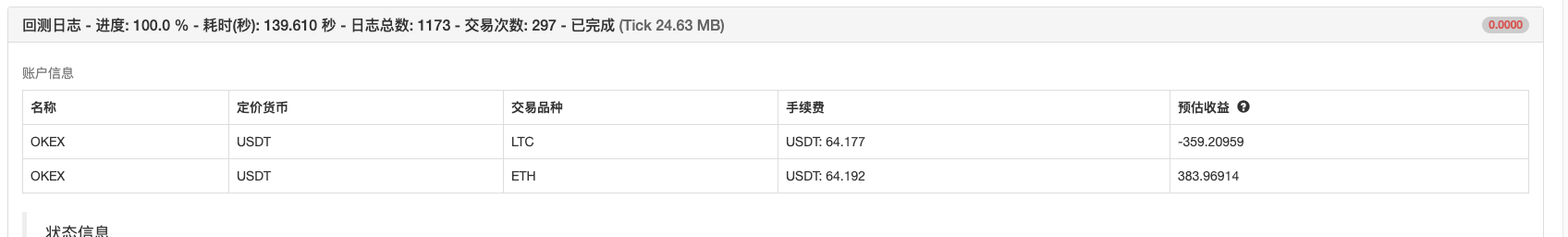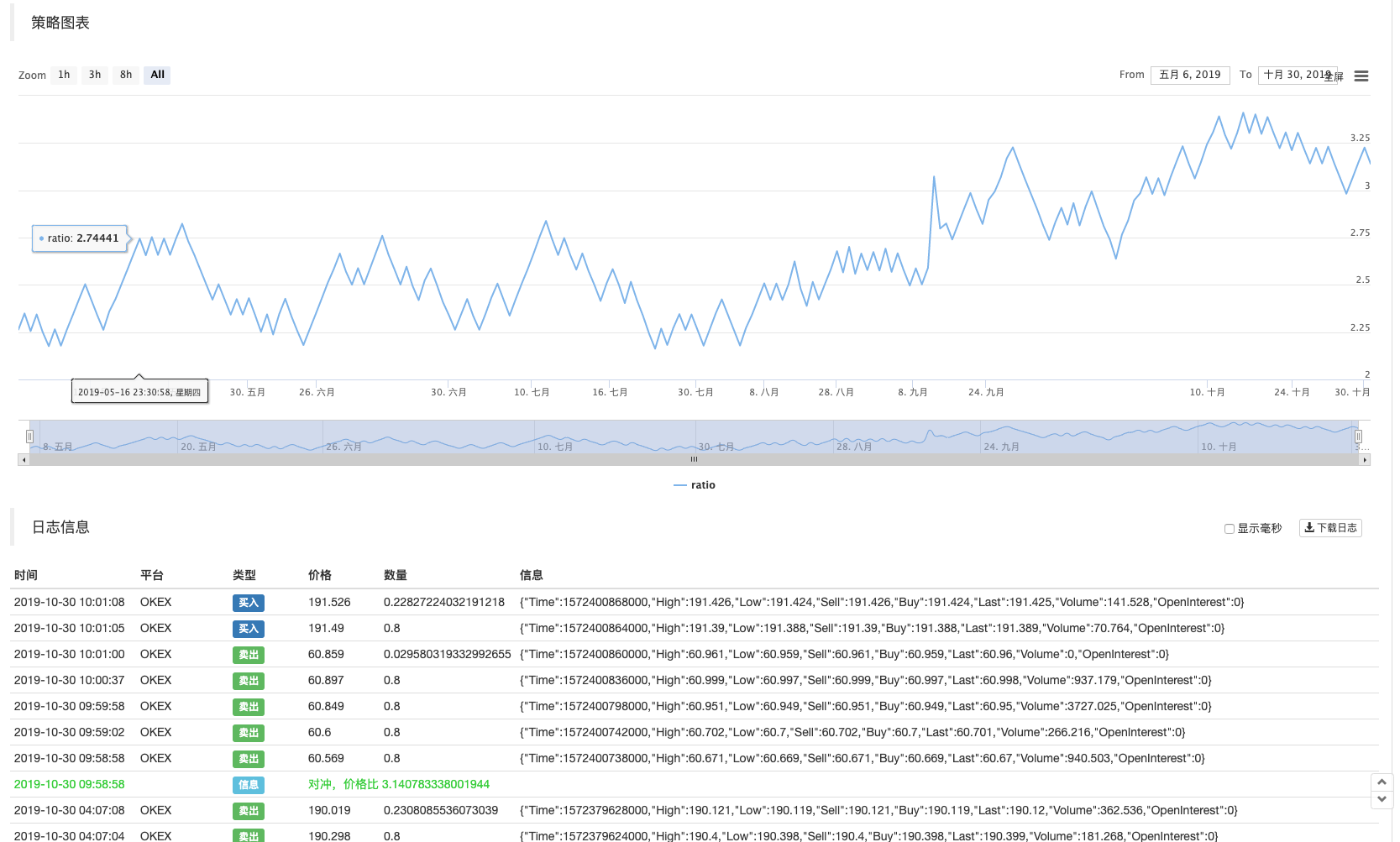# 区块链资产量化交易中的跨币种对冲策略

1年前   阅读数 100 0

A交易对为：LTC_USDT
B交易对为：ETH_USDT

「画线类库」：https://www.fmz.com/strategy/27293
「数字货币现货交易类库」：这个是每个用户新建策略时，模板栏中自带的。

``````/*backtest
start: 2019-05-01 00:00:00
end: 2019-11-04 00:00:00
period: 1m
exchanges: [{"eid":"OKEX","currency":"LTC_USDT","balance":100000,"stocks":30},{"eid":"OKEX","currency":"ETH_USDT","balance":100000,"stocks":30}]
*/

/*
A exchanges : EOS_USDT
B exchanges : ETH_USDT
*/

var Interval = 500

// 参数
var numPoint = 100        // 节点数
var distance = 0.08       // 比例间距
var amountPoint = 100     // 节点金额，单位USDT
var arrHedgeList = []

function main () {
var isFirst = true
while(true) {
var rA = exchanges.Go("GetTicker")
var rB = exchanges.Go("GetTicker")

var tickerA = rA.wait()
var tickerB = rB.wait()

if (tickerA && tickerB) {
var priceRatioSell = tickerB.Buy / tickerA.Sell     // B sell , A buy
var priceRatioBuy = tickerB.Sell / tickerA.Buy      // B buy , A sell

if (isFirst) {
for (var i = 0 ; i < numPoint ; i++) {
var point = {
priceRatio : priceRatioSell + (i + 1) * distance,
coverRatio : priceRatioSell + i * distance,
amount : (0.08 * i + 1) * amountPoint,
isHold : false,
}
arrHedgeList.push(point)
}
isFirst = false
}

for (var j = 0 ; j < arrHedgeList.length; j++) {
if (priceRatioSell > arrHedgeList[j].priceRatio && arrHedgeList[j].isHold == false) {
// B sell , A buy
Log("对冲，价格比", priceRatioSell, "#FF0000")
\$.Buy(exchanges, arrHedgeList[j].amount / tickerA.Sell)
\$.Sell(exchanges, arrHedgeList[j].amount / tickerB.Buy)
arrHedgeList[j].isHold = true
LogStatus(_D(), exchanges.GetAccount(), "\n", exchanges.GetAccount())
\$.PlotLine("ratio", (priceRatioSell + priceRatioBuy) / 2)
break
}

if (priceRatioBuy < arrHedgeList[j].coverRatio && arrHedgeList[j].isHold == true) {
// B buy , A sell
Log("对冲，价格比", priceRatioBuy, "#32CD32")
\$.Sell(exchanges, arrHedgeList[j].amount / tickerA.Buy)
\$.Buy(exchanges, arrHedgeList[j].amount / tickerB.Sell)
arrHedgeList[j].isHold = false
LogStatus(_D(), exchanges.GetAccount(), "\n", exchanges.GetAccount())
\$.PlotLine("ratio", (priceRatioSell + priceRatioBuy) / 2)
break
}
}
}
Sleep(Interval)
}
}
``````

### 可以通过回测，来最初步的验证一下策略思路``````if (isFirst) {
for (var i = 0 ; i < numPoint ; i++) {
var point = {
priceRatio : priceRatioSell + (i + 1) * distance,
coverRatio : priceRatioSell + i * distance,
amount : (0.08 * i + 1) * amountPoint,          // 每次递增amountPoint的8%
isHold : false,
}
arrHedgeList.push(point)
}
isFirst = false
}
``````

我有话说: